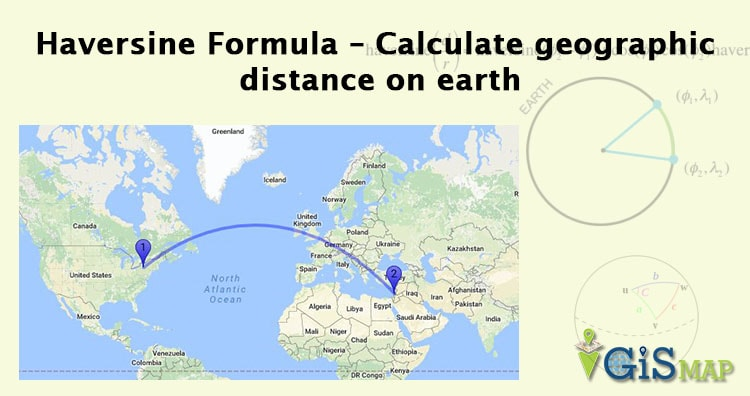# Haversine Formula – Calculate geographic distance on earthHaversine Formula – Calculate geographic distance on earth. If you have two different latitude – longitude values of two different point on earth, then with the help of Haversine Formula, you can easily compute the great-circle distance (The shortest distance between two points on the surface of a Sphere). The term Haversine was coined by Prof. James Inman in 1835. Haversine is very popular and frequently used formula when developing a GIS (Geographic Information System) application or analyzing path and fields.

## Haversine formula:

Central angle Haversine can be computed, between two points with r as radius of earth, d as the distance between two points,$\phi_1, \phi_2$ is latitude of two points and$\lambda_1, \lambda_2$ is longitude of two points respectively, as:$\operatorname{haversin}\left(\frac{d}{r}\right) = \operatorname{haversin}(\phi_2 - \phi_1) + \cos(\phi_1) \cos(\phi_2)\operatorname{haversin}(\lambda_2-\lambda_1)$

### Law of Haversine:

To derive law of Haversine one needs to start the calculation with spherical law of cosine i.e cos a = cos b * cos c + sin b * sin c * cos A

One can derive Haversine formula to calculate distance between two as:

a = sin²(ΔlatDifference/2) + cos(lat1).cos(lt2).sin²(ΔlonDifference/2)
c = 2.atan2(√a, √(1−a))
d = R.c

where,

ΔlatDifference = lat1 – lat2 (difference of latitude)

ΔlonDifference = lon1 – lon2 (difference of longitude)

R is radius of earth i.e 6371 KM or 3961 miles

and d is the distance computed between two points.

### Here is the example result delivered by Haversine Formula:

Lets take one of latitude-longitude for calculation distance,
NEBRASKA, USA (Latitude : 41.507483, longitude : -99.436554) and
KANSAS, USA (Latitude : 38.504048, Longitude : -98.315949)

Do compute the distance with the above written formula. Your answer would be 347.3 Km(kilo-meter). The snapshot demonstrate the same result with map below.